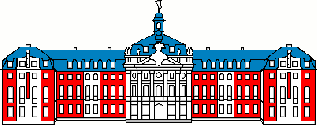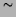Next: Contents   ContentsUniversität Münster

Institut für Theoretische Physik I

Bayesian Field Theory
Nonparametric Approaches to Density Estimation, Regression, Classification, and Inverse Quantum Problems

Jörg C. Lemm

This publication can be retrieved from the URL: http://pauli.uni-muenster.de/lemm/

Email: lemm@uni-muenster.de

# Bayesian Field TheoryNonparametric Approaches to Density Estimation, Regression, Classification, and Inverse Quantum Problems

Jörg C. Lemm1

Institut für Theoretische Physik I
Universität Münster
Wilhelm-Klemm-Str.9
D-48149 Münster, Germany

### Abstract:

Bayesian field theory denotes a nonparametric Bayesian approach for learning functions from observational data. Based on the principles of Bayesian statistics, a particular Bayesian field theory is defined by combining two models: a likelihood model, providing a probabilistic description of the measurement process, and a prior model, providing the information necessary to generalize from training to non-training data. The particular likelihood models discussed in the paper are those of general density estimation, Gaussian regression, clustering, classification, and models specific for inverse quantum problems. Besides problem typical hard constraints, like normalization and non-negativity for probabilities, prior models have to implement all the specific, and often vague, a priori knowledge available for a specific task. Nonparametric prior models discussed in the paper are Gaussian processes, mixtures of Gaussian processes, and non-quadratic potentials. Prior models are made flexible by including hyperparameters. In particular, the adaption of mean functions and covariance operators of Gaussian process components is discussed in detail. Even if constructed using Gaussian process building blocks, Bayesian field theories are typically non-Gaussian and have thus to be solved numerically. According to increasing computational resources the class of non-Gaussian Bayesian field theories of practical interest which are numerically feasible is steadily growing. Models which turn out to be computationally too demanding can serve as starting point to construct easier to solve parametric approaches, using for example variational techniques.Next: Contents   Contents
Joerg_Lemm 2001-01-21